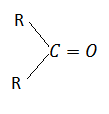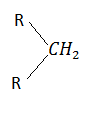The shortest $\mathrm{C}-\mathrm{C}$ bond distance is found in

(a) diamond                                 (b) ethane

(c) benzene                                  (d) acetylene

Concept Questions :-

Aliphatic Hydrocarbon- physical properties
High Yielding Test Series + Question Bank - NEET 2020

Difficulty Level:

Benzene reacts with n-propyl chloride in the presence of anhydrous ${\mathrm{AlCl}}_{3}$ to give

(a) 3-propyl-1-chlorobenzene

(c) n-propyl benzene

(c) no reaction

(d) iso-propyl benzene

Concept Questions :-

Aromatic Hydrocarbons - Benzene - Structure, Preparation & Chemical Reactions
High Yielding Test Series + Question Bank - NEET 2020

Difficulty Level:

The distance between two adjacent cabron atoms is largest in

(a) benzene                              (b) ethene

(c) butane                                (d) ethyne

Concept Questions :-

Aliphatic Hydrocarbon- physical properties
High Yielding Test Series + Question Bank - NEET 2020

Difficulty Level:

In benzene, $\mathrm{C}-\mathrm{C}$ bond length is 1.39 $\stackrel{°}{\mathrm{A}}$; the $\mathrm{C}-\mathrm{H}$ bond length is:

1. 1.39                                     2. 1.08

3. 1.54                                     4. 1.46

Concept Questions :-

Aliphatic Hydrocarbon- physical properties
High Yielding Test Series + Question Bank - NEET 2020

Difficulty Level:

Which reaction produces acrylonitrile (${\mathrm{CH}}_{2}=\mathrm{CHCN}$)?

1. Ethyne $\underset{{\mathrm{Ba}}^{2+}}{\overset{\mathrm{HCN}}{\to }}$                           2. Acrylic acid $\stackrel{\mathrm{KCN}}{\to }$

3. Ethyne $\stackrel{\mathrm{KCN}}{\to }$                           4. Ethyne $\stackrel{\mathrm{HOCl}}{\to }$

Concept Questions :-

Alkanes, Alkenes and Alkynes - Chemical Properties
High Yielding Test Series + Question Bank - NEET 2020

Difficulty Level:is not converted toby:

(a) Wolff-Kishner reaction

(b) Clemmensen reduction

(c) red P + HI at $200°\mathrm{C}$

(d) Wurtz's reaction

Concept Questions :-

Alkanes, Alkenes and Alkynes - Chemical Properties
High Yielding Test Series + Question Bank - NEET 2020

Difficulty Level:

Products of the following reaction

... are

1. ${\mathrm{CH}}_{3}\mathrm{CHO}$ + ${\mathrm{CH}}_{3}{\mathrm{CH}}_{2}\mathrm{CHO}$

2. ${\mathrm{CH}}_{3}\mathrm{COOH}$ + ${\mathrm{CH}}_{3}{\mathrm{COCH}}_{3}$

3. ${\mathrm{CH}}_{3}\mathrm{COOH}$$\mathrm{HOOC}.{\mathrm{CH}}_{2}{\mathrm{CH}}_{3}$

4. ${\mathrm{CH}}_{3}\mathrm{COOH}$${\mathrm{CO}}_{2}$

Concept Questions :-

Alkanes, Alkenes and Alkynes - Chemical Properties
High Yielding Test Series + Question Bank - NEET 2020

Difficulty Level:

Acetylene reacts with 42% ${\mathrm{H}}_{2}{\mathrm{SO}}_{4}$ containing 1% ${\mathrm{HgSO}}_{4}$ to give:

1. ${\mathrm{C}}_{2}{\mathrm{H}}_{5}{\mathrm{HSO}}_{4}$                               2. ${\mathrm{CH}}_{3}\mathrm{CHO}$

3. HCHO                                       4. ${\mathrm{CH}}_{2}={\mathrm{CH}}_{2}$

Concept Questions :-

Alkanes, Alkenes and Alkynes - Chemical Properties
High Yielding Test Series + Question Bank - NEET 2020

Difficulty Level:

When ${\mathrm{CaC}}_{2}$ was hydrolysed a gas was obtained. It had a garlic odour due to phosgene present as impurity. The gas was passed through ammoniacal solution of ${\mathrm{Cu}}_{2}{\mathrm{Cl}}_{2}$, a red ppt. was obtained. The gas was:

1. ethylene                                       2. propyne

3. acetylene                                      4. ethane

Concept Questions :-

Alkanes, Alkenes and Alkynes - Chemical Properties
High Yielding Test Series + Question Bank - NEET 2020

Difficulty Level:

Which of the following compounds with molecular formula, ${\mathrm{C}}_{5}{\mathrm{H}}_{10}$ yields acetone on ozonolysis?

(a) 2-methyl-2-butene

(b) 3-methyl-1-butene

(c) Cyclopentane

(d) 2-methyl-1-butene

Concept Questions :-

Alkanes, Alkenes and Alkynes - Chemical Properties